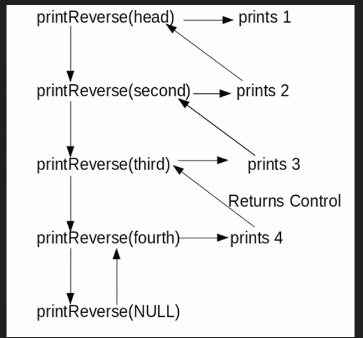# Java Program For Printing Reverse Of A Linked List Without Actually Reversing

• Last Updated : 13 Dec, 2021

Given a linked list, print reverse of it using a recursive function. For example, if the given linked list is 1->2->3->4, then output should be 4->3->2->1.
Note that the question is only about printing the reverse. To reverse the list itself see this
Difficulty Level: RookieAlgorithm:

```printReverse(head)
1. call print reverse for head->next

Implementation:

## Java

 `// Java program to print reverse ``// of a linked list``class` `LinkedList``{``    ``// Head of list``    ``Node head;  `` ` `    ``// Linked list Node``    ``class` `Node``    ``{``        ``int` `data;``        ``Node next;``        ``Node(``int` `d) ``        ``{``            ``data = d; ``            ``next = ``null``; ``        ``}``    ``}`` ` `    ``// Function to print reverse of ``    ``// linked list ``    ``void` `printReverse(Node head)``    ``{``        ``if` `(head == ``null``) ``return``;`` ` `        ``// Print list of head node``        ``printReverse(head.next);`` ` `        ``// After everything else is printed,``        ``//  Print head``        ``System.out.print(head.data + ``" "``);``    ``}`` ` `    ``// Utility Functions `` ` `    ``// Inserts a new Node at front ``    ``// of the list. ``    ``public` `void` `push(``int` `new_data)``    ``{``        ``/* 1 & 2: Allocate the Node &``                  ``Put in the data*/``        ``Node new_node = ``new` `Node(new_data);`` ` `        ``// 3. Make next of new Node as head ``        ``new_node.next = head;`` ` `        ``// 4. Move the head to point ``        ``// to new Node ``        ``head = new_node;``    ``}`` ` `    ``// Driver code``    ``public` `static` `void` `main(String args[])``    ``{``        ``// Create linked list 1->2->3->4``        ``LinkedList llist = ``new` `LinkedList();``        ``llist.push(``4``);``        ``llist.push(``3``);``        ``llist.push(``2``);``        ``llist.push(``1``);`` ` `        ``llist.printReverse(llist.head);``    ``}``}``// This code is contributed by Rajat Mishra `

Output:

`4 3 2 1`

Time Complexity: O(n)

Please refer complete article on Print reverse of a Linked List without actually reversing for more details!

My Personal Notes arrow_drop_up